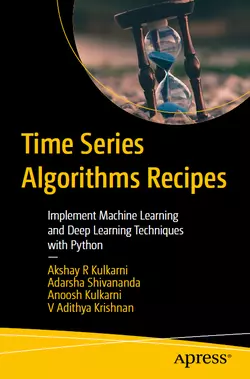# Time Series Algorithms Recipes### eBook Details:

• Paperback: 190 pages
• Publisher: WOW! eBook (January 7, 2023)
• Language: English
• ISBN-10: 1484289773
• ISBN-13: 978-1484289778

### eBook Description:

Time Series Algorithms Recipes: Implement Machine Learning and Deep Learning Techniques with Python

This book teaches the practical implementation of various concepts for time series analysis and modeling with Python through problem-solution-style recipes, starting with data reading and preprocessing.

It begins with the fundamentals of time series forecasting using statistical modeling methods like AR (autoregressive), MA (moving-average), ARMA (autoregressive moving-average), and ARIMA (autoregressive integrated moving-average). Next, you’ll learn univariate and multivariate modeling using different open-sourced packages like Fbprohet, stats model, and sklearn. You’ll also gain insight into classic machine learning-based regression models like randomForest, Xgboost, and LightGBM for forecasting problems. The book concludes by demonstrating the implementation of deep learning models (LSTMs and ANN) for time series forecasting. Each chapter includes several code examples and illustrations.

• Implement various techniques in time series analysis using Python
• Utilize statistical modeling methods such as AR (autoregressive), MA (moving-average), ARMA (autoregressive moving-average) and ARIMA (autoregressive integrated moving-average) for time series forecasting
• Understand univariate and multivariate modeling for time series forecasting
• Forecast using machine learning and deep learning techniques such as GBM and LSTM (long short-term memory)

After finishing this Time Series Algorithms Recipes book, you will have a foundational understanding of various concepts relating to time series and its implementation in Python.

Exclusive Offer! Order 2 in 1 Home Sink Organizer Now. Get Lowest Price & 60 Day Return Policy. Huge Discounts Available! Bravo Goods Special Offer Expires Soon.